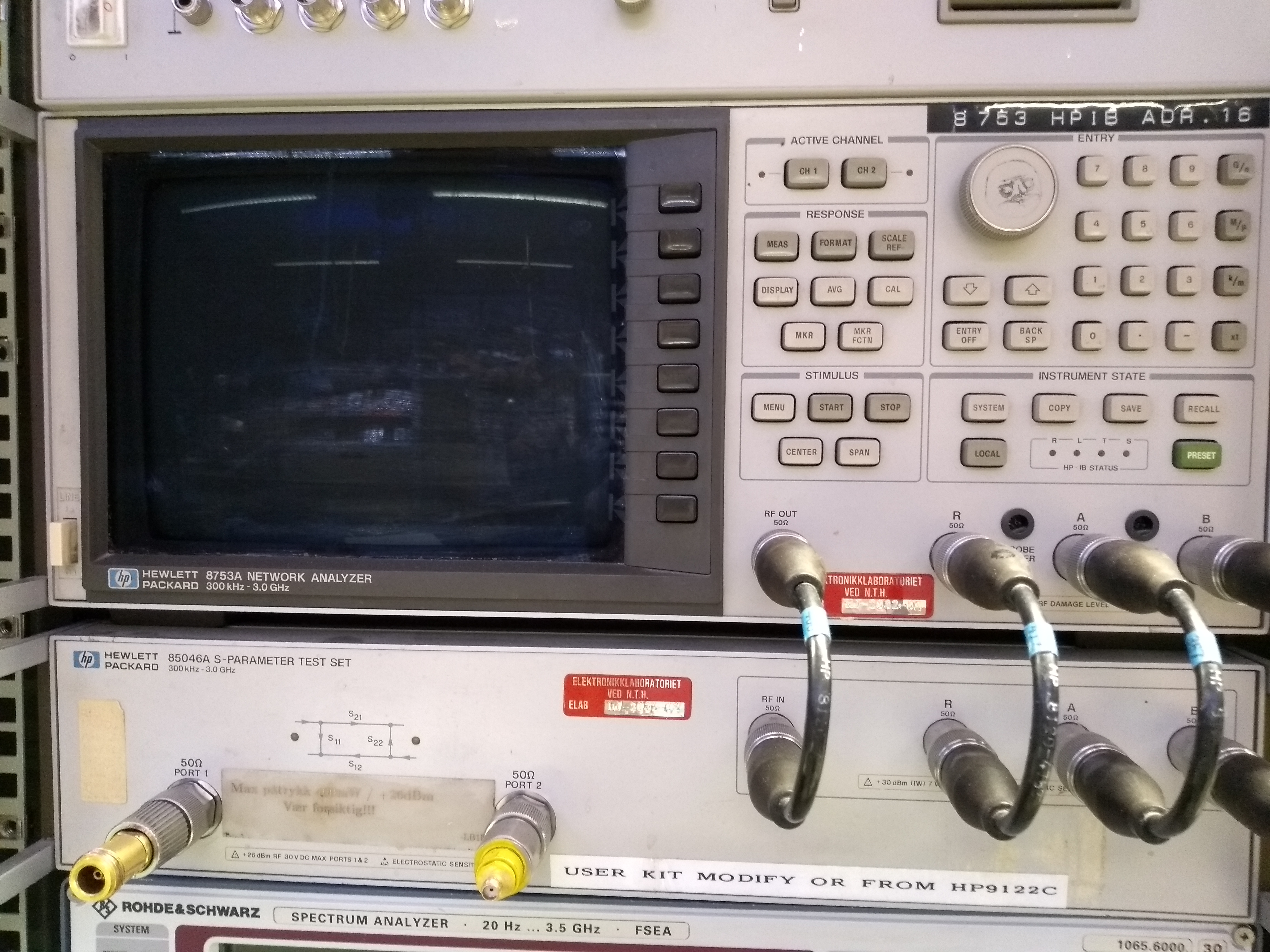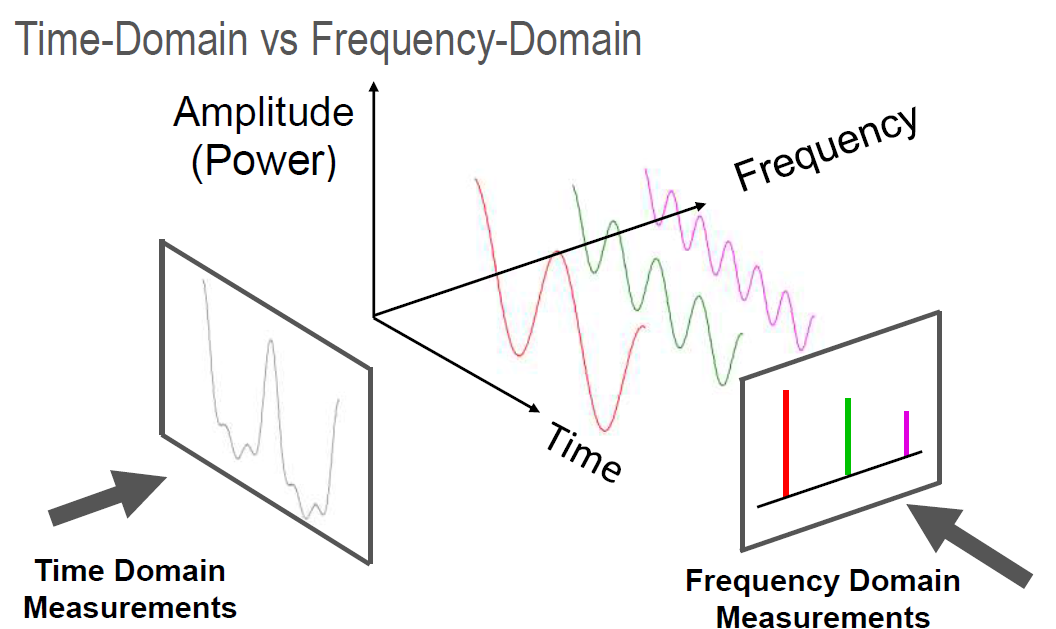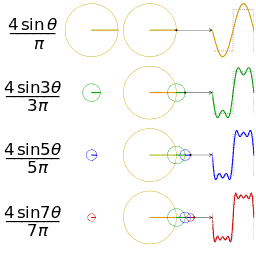##### Page tree
Go to start of banner

# Network Analyzer

A Network Analyzer is used to measure the impedance across its terminals, for instance in an RF circuit or a resonance circuit such as an antenna. The circuit being measured on is called a network. Since impedance is frequency dependent, the instrument sweeps across a range of frequencies. The instrument inputs a signal of the given frequency into the network, and measures the response. The same operation is repeated for many different (a spectrum of) frequencies. This is called a frequency sweep

It measures S-parameters.

# HP8753A

300kHz - 3.0GHz two port network analyzer.### User Guide & Service ManualThe real magical gotcha of frequency domain analysis is that any function can be represented as an infinite sum of sine waves. That means that whatever signal you have in the left frame, you can get an arbitrarily close approximation of it by summing enough sinus signals. For example, if we want to get a square wave:###### (Figure from Wikimedia Commons)

If you zoom in on the corners of a square wave on a good oscilloscope, you will see this effect. This is because your signal passes through components that cannot support infinitely high frequencies, so that a square wave cannot be perfectly represented.

Simply put, frequency analysis represents a signal as the magnitudes of the sine waves required to "build" it.

• No labels